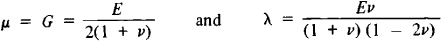Lamé Constants

Lamé constants

[lä′mā ‚kän·stəns]
(mechanics)
Two constants which relate stress to strain in an isotropic, elastic material.
McGraw-Hill Dictionary of Scientific & Technical Terms, 6E, Copyright © 2003 by The McGraw-Hill Companies, Inc.
The following article is from The Great Soviet Encyclopedia (1979). It might be outdated or ideologically biased.

Lamé Constants

values that relate the components of elastic stress at some point of a solid isotropic deformable body to the components of strain at this point:

σx = 2μεxx + λ(εxx + εyy + εzz)

τ = μεxy

where σ and τ are the normal and tangential components of stress, ε are the components of strain, and the coefficients λ and μ are Lamé constants, named after the French mathematician G. Lamé. The Lamé constants depend on the material and its temperature. They are related to the modulus of elasticity (Young’s modulus) and Poisson’s ratio v bywhere E is the modulus of elasticity and G is the shear modulus.